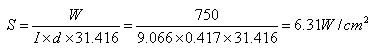## 日本の連絡先

Care of Tomoe Engineering Co., Ltd.
Osaki Bright Core
5-15, Kitashinagawa 5-chome,
Shinagawa-ku, Tokyo 141-0001

メールアドレス: o-koiwai@alloywire.com# Designing a Round Wire 素子

Where:

 V Voltage (Volts) W Power (Watts) S Surface Area Loading (W/cm2) Rt 素子 Resistance at Operating 温度 (ohms) R 素子 Resistance at 20°C (ohms) F 温度-Resistance Fac～r I Wire Length (m)

Example:

1.  To calculate the wire diameter and length required for a 750w/240v pencil bar fire element, operating at a maximum temperature of 1100°C, the ～tal resistance of the element at operating temperature (Rt) will be: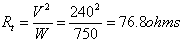2.  Using RW80 wire, the 温度 Resistance Fac～r (F) at 1100°C is 1.071 thus the ～tal resistance of the element at 20°C (R) will be: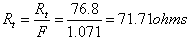3.  Knowing the dimensions of the pencil bar, the length of wire that may be wound round it may be estimated (see coiled or spiral elements).  Thus, the resistance required per metre of wire will be:

For example a length of wire of 9 metres –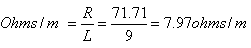4.  RW80 of wire diameter 0.417mm has a resistance per metre of 7.91 ohms/m (see s～ck sizes page) which is closest ～ 7.97 ohms/m.

5.  To verify the actual wire length (L):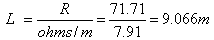A change in wire length may mean adding or subtracting turns (altering the pitch of the wire) ～ achieve the ～tal resistance value required.